# Bridges SDE’s

Consider now a $$d$$-dimensional stochastic process $$X_{t}$$ defined on a probability space $$(\Omega, \mathfrak{F},\mathbb{P})$$. We say that the bridge associated to $$X_{t}$$ conditioned to the event $$\{X_{T}= a\}$$ is the process: $\{\tilde{X}_{t}, t_{0} \leq t \leq T \}=\{X_{t}, t_{0} \leq t \leq T: X_{T}= a \}$ where $$T$$ is a deterministic fixed time and $$a \in \mathbb{R}^d$$ is fixed too.

# The bridgesdekd() functions

The (S3) generic function bridgesdekd() (where k=1,2,3) for simulation of 1,2 and 3-dim bridge stochastic differential equations,Ito or Stratonovich type, with different methods. The main arguments consist:

• The drift and diffusion coefficients as R expressions that depend on the state variable x (y and z) and time variable t.
• corr the correlation structure of standard Wiener process ; must be a real symmetric positive-definite square matrix (2D and 3D, default: corr=NULL).
• The number of simulation steps N.
• The number of the solution trajectories to be simulated by M (default: M=1).
• Initial value x0 at initial time t0.
• Terminal value y final time T
• The integration step size Dt (default: Dt=(T-t0)/N).
• The choice of process types by the argument type="ito" for Ito or type="str" for Stratonovich (by default type="ito").
• The numerical method to be used by method (default method="euler").

By Monte-Carlo simulations, the following statistical measures (S3 method) for class bridgesdekd() (where k=1,2,3) can be approximated for the process at any time $$t \in [t_{0},T]$$ (default: at=(T-t0)/2):

• The expected value $$\text{E}(X_{t})$$ at time $$t$$, using the command mean.
• The variance $$\text{Var}(X_{t})$$ at time $$t$$, using the command moment with order=2 and center=TRUE.
• The median $$\text{Med}(X_{t})$$ at time $$t$$, using the command Median.
• The mode $$\text{Mod}(X_{t})$$ at time $$t$$, using the command Mode.
• The quartile of $$X_{t}$$ at time $$t$$, using the command quantile.
• The maximum and minimum of $$X_{t}$$ at time $$t$$, using the command min and max.
• The skewness and the kurtosis of $$X_{t}$$ at time $$t$$, using the command skewness and kurtosis.
• The coefficient of variation (relative variability) of $$X_{t}$$ at time $$t$$, using the command cv.
• The central moments up to order $$p$$ of $$X_{t}$$ at time $$t$$, using the command moment.
• The result summaries of the results of Monte-Carlo simulation at time $$t$$, using the command summary.

We can just make use of the rsdekd() function (where k=1,2,3) to build our random number for class bridgesdekd() (where k=1,2,3) at any time $$t \in [t_{0},T]$$. the main arguments consist:

• object an object inheriting from class bridgesdekd() (where k=1,2,3).
• at time between $$s=t0$$ and $$t=T$$.

The function dsde() (where k=1,2,3) approximate transition density for class bridgesdekd() (where k=1,2,3), the main arguments consist:

• object an object inheriting from class bridgesdekd() (where k=1,2,3).
• at time between $$s=t0$$ and $$t=T$$.
• pdf probability density function Joint or Marginal.

The following we explain how to use this functions.

# bridgesde1d()

Assume that we want to describe the following bridge sde in Ito form: $\begin{equation}\label{eq0166} dX_t = \frac{1-X_t}{1-t} dt + X_t dW_{t},\quad X_{t_{0}}=3 \quad\text{and}\quad X_{T}=1 \end{equation}$ We simulate a flow of $$1000$$ trajectories, with integration step size $$\Delta t = 0.001$$, and $$x_0 = 3$$ at time $$t_0 = 0$$, $$y = 1$$ at terminal time $$T=1$$.

R> set.seed(1234, kind = "L'Ecuyer-CMRG")
R> f <- expression((1-x)/(1-t))
R> g <- expression(x)
R> mod <- bridgesde1d(drift=f,diffusion=g,x0=3,y=1,M=1000)
R> mod
Itô Bridge Sde 1D:
| dX(t) = (1 - X(t))/(1 - t) * dt + X(t) * dW(t)
Method:
| Euler scheme with order 0.5
Summary:
| Size of process   | N = 1001.
| Crossing realized | C = 978 among 1000.
| Initial value     | x0 = 3.
| Ending value      | y = 1.
| Time of process   | t in [0,1].
| Discretization    | Dt = 0.001.

In Figure 1, we present the flow of trajectories, the mean path (red lines) of solution of $$X_{t}|X_{0}=3,X_{T}=1$$:

R> plot(mod,ylab=expression(X[t]))
R> lines(time(mod),apply(mod\$X,1,mean),col=2,lwd=2)
R> legend("topleft","mean path",inset = .01,col=2,lwd=2,cex=0.8,bty="n")

Hence we can just make use of the rsde1d() function to build our random number generator for $$X_{t}|X_{0}=3,X_{T}=1$$ at time $$t=0.55$$:

R> x <- rsde1d(object = mod, at = 0.55)
R> head(x, n = 3)
 0.72282 0.96118 0.94990

The function dsde1d() can be used to show the kernel density estimation for $$X_{t}|X_{0}=3,X_{T}=1$$ at time $$t=0.55$$ (hist=TRUE based on truehist() function in MASS package):

R> dens <- dsde1d(mod, at = 0.55)
R> plot(dens,hist=TRUE) ## histgramme
R> plot(dens,add=TRUE)  ## kernel density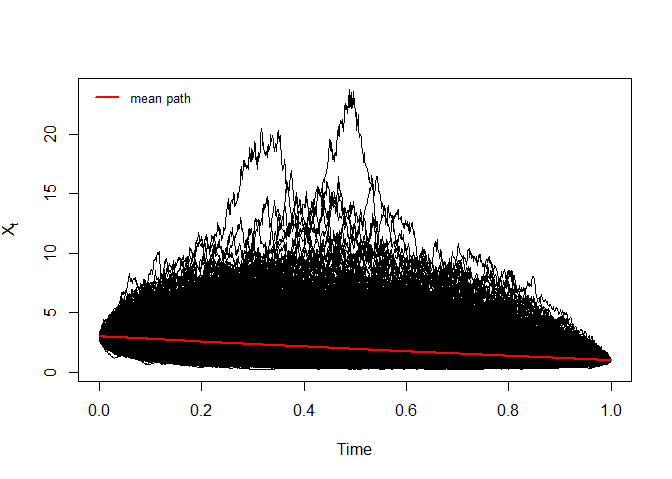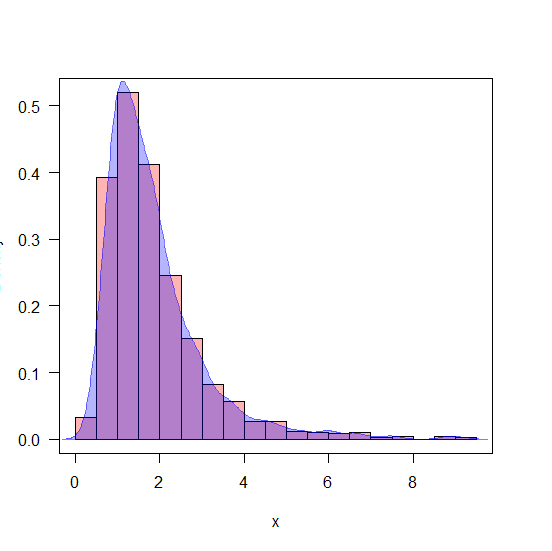# bridgesde2d()

Assume that we want to describe the following $$2$$-dimensional bridge SDE’s in Stratonovich form:

$\begin{equation}\label{eq:09} \begin{cases} dX_t = -(1+Y_{t}) X_{t} dt + 0.2 (1-Y_{t})\circ dB_{1,t},\quad X_{t_{0}}=1 \quad\text{and}\quad X_{T}=1\\ dY_t = -(1+X_{t}) Y_{t} dt + 0.1 (1-X_{t}) \circ dB_{2,t},\quad Y_{t_{0}}=-0.5 \quad\text{and}\quad Y_{T}=0.5 \end{cases} \end{equation}$ with $$(B_{1,t},B_{2,t})$$ are two correlated standard Wiener process: $\Sigma= \begin{pmatrix} 1 & 0.3\\ 0.3 & 1 \end{pmatrix}$

We simulate a flow of $$1000$$ trajectories, with integration step size $$\Delta t = 0.01$$:

R> set.seed(1234, kind = "L'Ecuyer-CMRG")
R> fx <- expression(-(1+y)*x , -(1+x)*y)
R> gx <- expression(0.2*(1-y),0.1*(1-x))
R> Sigma <-matrix(c(1,0.3,0.3,1),nrow=2,ncol=2)
R> mod2 <- bridgesde2d(drift=fx,diffusion=gx,x0=c(1,-0.5),y=c(1,0.5),Dt=0.01,M=1000,type="str",corr=Sigma)
R> mod2
Stratonovich Bridge Sde 2D:
| dX(t) = -(1 + Y(t)) * X(t) * dt + 0.2 * (1 - Y(t)) o dB1(t)
| dY(t) = -(1 + X(t)) * Y(t) * dt + 0.1 * (1 - X(t)) o dB2(t)
| Correlation structure:
1.0 0.3
0.3 1.0
Method:
| Euler scheme with order 0.5
Summary:
| Size of process   | N = 1001.
| Crossing realized | C = 1000 among 1000.
| Initial values    | x0 = (1,-0.5).
| Ending values     | y = (1,0.5).
| Time of process   | t in [0,10].
| Discretization    | Dt = 0.01.

In Figure 2, we present the flow of trajectories of $$X_{t}|X_{0}=1,X_{T}=1$$ and $$Y_{t}|Y_{0}=-0.5,Y_{T}=0.5$$:

R> plot(mod2,col=c('#FF00004B','#0000FF82'))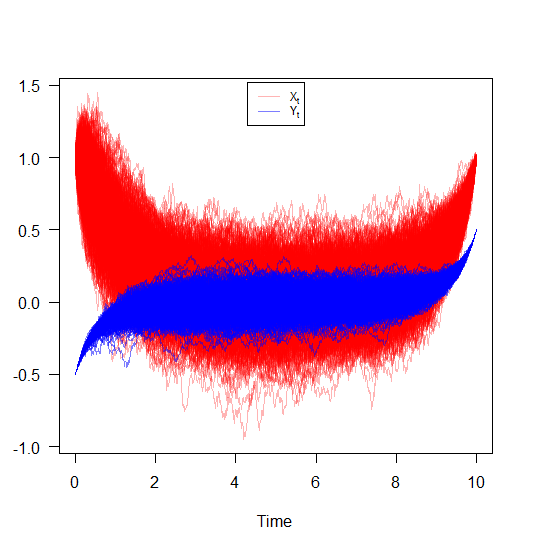Bridge sde 2D

Hence we can just make use of the rsde2d() function to build our random number generator for the couple $$X_{t},Y_{t}|X_{0}=1,Y_{0}=-0.5,X_{T}=1,Y_{T}=0.5$$ at time $$t=5$$:

R> x2 <- rsde2d(object = mod2, at = 5)
R> head(x2, n = 3)
         x          y
1 0.051742  0.0095811
2 0.135792  0.0436799
3 0.021494 -0.0348084

The marginal density of $$X_{t}|X_{0}=1,X_{T}=1$$ and $$Y_{t}|Y_{0}=-0.5,Y_{T}=0.5$$ at time $$t=5$$ are reported using dsde2d() function. A contour plot of joint density obtained from a realization of the couple $$X_{t},Y_{t}|X_{0}=1,Y_{0}=-0.5,X_{T}=1,Y_{T}=0.5$$ at time $$t=5$$, see e.g. Figure 3:

R> ## Marginal
R> denM <- dsde2d(mod2,pdf="M",at = 5)
R> plot(denM, main="Marginal Density")
R> ## Joint
R> denJ <- dsde2d(mod2, pdf="J", n=100,at = 5)
R> plot(denJ,display="contour",main="Bivariate Transition Density at time t=5")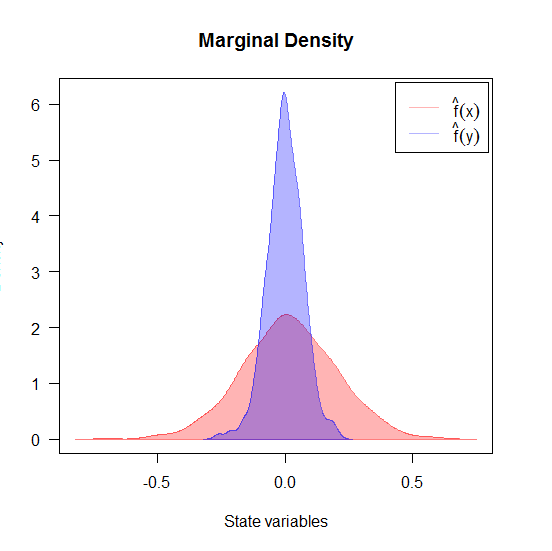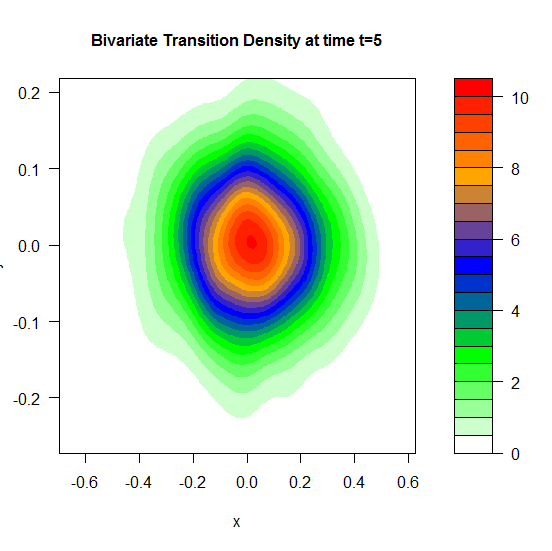A $$3$$D plot of the transition density at $$t=5$$ obtained with:

R> plot(denJ,main="Bivariate Transition Density at time t=5")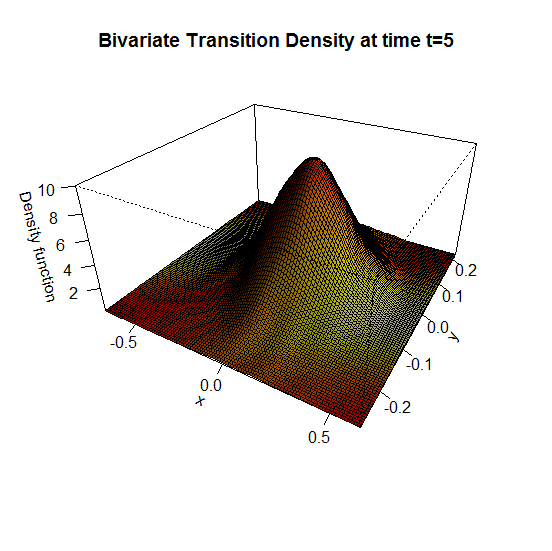$$3$$D plot of the transition density of $$X_{t}|X_{0}=1,X_{T}=1$$ and $$Y_{t}|Y_{0}=-0.5,Y_{T}=0.5$$ at time $$t=5$$

We approximate the bivariate transition density over the set transition horizons $$t\in [1,9]$$ with $$\Delta t = 0.005$$ using the code:

R> for (i in seq(1,9,by=0.005)){
+ plot(dsde2d(mod2, at = i,n=100),display="contour",main=paste0('Transition Density \n t = ',i))
+ }

# bridgesde3d()

Assume that we want to describe the following bridges SDE’s (3D) in Ito form:

$\begin{equation} \begin{cases} dX_t = -4 (1+X_{t}) Y_{t} dt + 0.2 dW_{1,t},\quad X_{t_{0}}=0 \quad\text{and}\quad X_{T}=0\\ dY_t = 4 (1-Y_{t}) X_{t} dt + 0.2 dW_{2,t},\quad Y_{t_{0}}=-1 \quad\text{and}\quad Y_{T}=-2\\ dZ_t = 4 (1-Z_{t}) Y_{t} dt + 0.2 dW_{3,t},\quad Z_{t_{0}}=0.5 \quad\text{and}\quad Z_{T}=0.5 \end{cases} \end{equation}$

We simulate a flow of $$1000$$ trajectories, with integration step size $$\Delta t = 0.001$$.

R> set.seed(1234, kind = "L'Ecuyer-CMRG")
R> fx <- expression(-4*(1+x)*y, 4*(1-y)*x, 4*(1-z)*y)
R> gx <- rep(expression(0.2),3)
R> mod3 <- bridgesde3d(x0=c(0,-1,0.5),y=c(0,-2,0.5),drift=fx,diffusion=gx,M=1000)
R> mod3
Itô Bridge Sde 3D:
| dX(t) = -4 * (1 + X(t)) * Y(t) * dt + 0.2 * dW1(t)
| dY(t) = 4 * (1 - Y(t)) * X(t) * dt + 0.2 * dW2(t)
| dZ(t) = 4 * (1 - Z(t)) * Y(t) * dt + 0.2 * dW3(t)
Method:
| Euler scheme with order 0.5
Summary:
| Size of process   | N = 1001.
| Crossing realized | C = 998 among 1000.
| Initial values    | x0 = (0,-1,0.5).
| Ending values     | y  = (0,-2,0.5).
| Time of process   | t in [0,1].
| Discretization    | Dt = 0.001.

For plotting (back in time) using the command plot, and plot3D in space the results of the simulation are shown in Figure 5:

R> plot(mod3) ## in time
R> plot3D(mod3,display = "persp",main="3D Bridge SDE's") ## in space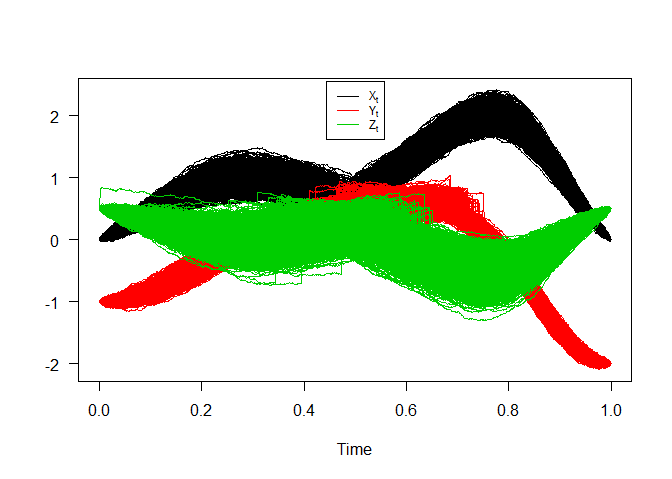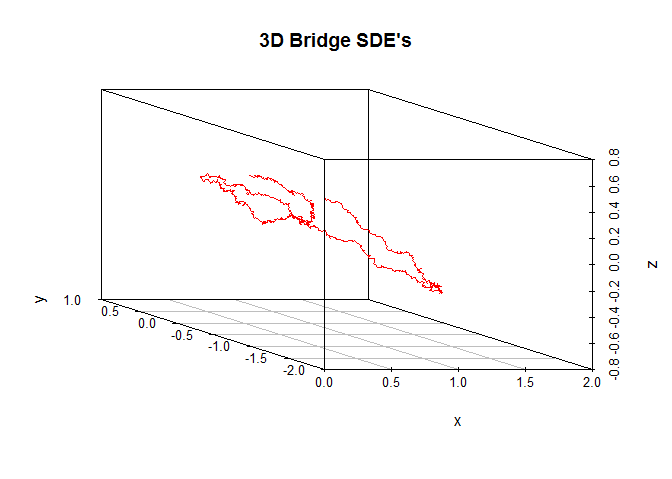Hence we can just make use of the rsde3d() function to build our random number generator for the triplet $$X_{t},Y_{t},Z_{t}|X_{0}=0,Y_{0}=-1,Z_{0}=0.5,X_{T}=0,Y_{T}=-2,Z_{T}=0.5$$ at time $$t=0.75$$:

R> x3 <- rsde3d(object = mod3, at = 0.75)
R> head(x3, n = 3)
       x        y        z
1 2.0636 0.074044 -0.30984
2 1.9445 0.111705 -0.26487
3 1.9288 0.054322 -0.50509

the density of $$X_{t}|X_{0}=0,X_{T}=0$$, $$Y_{t}|Y_{0}=-1,Y_{T}=-2$$ and $$Z_{t}|Z_{0}=0.5,Z_{T}=0.5$$ process at time $$t=0.75$$ are reported using dsde3d() function. For an approximate joint density for triplet $$X_{t},Y_{t},Z_{t}|X_{0}=0,Y_{0}=-1,Z_{0}=0.5,X_{T}=0,Y_{T}=-2,Z_{T}=0.5$$ at time $$t=0.75$$ (for more details, see package sm or ks.)

R> ## Marginal
R> denM <- dsde3d(mod3,pdf="M",at =0.75)
R> plot(denM, main="Marginal Density")
R> ## Joint
R> denJ <- dsde3d(mod3,pdf="J",at=0.75)
R> plot(denJ,display="rgl")